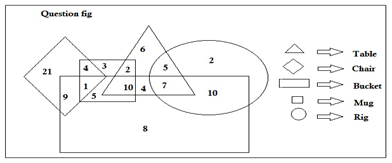# SSC CGL 2018 Practice Test Papers | Quantitative Aptitude (Day-5)

Dear Aspirants, Here we have given the Important SSC CGL Exam 2018 Practice Test Papers. Candidates those who are preparing for SSC CGL 2018 can practice these questions to get more confidence to Crack SSC CGL 2018 Examination.

[WpProQuiz 2670]

Click “Start Quiz” to attend these Questions and view Explanation

1. A train crosses a bridge in 45 seconds and a pole in 15 seconds. What is the Length of the train?

A. 300

B. 600

C. 450

D. Cannot be determined

2. Area of an equilateral triangle is 200√3 sq.cm. Find its side.

A. 20

B. 20√3

C. 20√2

D. 20√5

1. The number of prime numbers between 31 and 60 is

A. 9

B. 6

C. 8

D. 7

4. In the given figure, how many Buckets are there which is also Chair?A. 30

B. 10

C. 27

D. 16

5. In the given figure, how many Tables are there which are both bucket and Rig?A. 10

B. 17

C. 7

D. 12

1. In the given figure, how many mugs are there which are tables but not Chair?A.15

B.25

C.12

D.5

1. In the given figure, what is the sum of number of buckets and Rigs?A.56

B.73

C.61

D.55

1. The price of an article is increased by 5%.To restore its original price the new price must be decreased by

a.5.09

b.4.77

c.4.11

d.4.97

1. Unit digit of (164)102+ (164)103 =

a.1

b.6

c.0

d.4

1. The sum of square of two positive numbers is 149 and the square of sum of two positive numbers is 289.What is the product of two numbers?

a.140

b.70

c.210

d.438

Area of equilateral triangle= √3/4 a2

√3/4*a*a=200√3

a=20√2

The prime numbers between 31 and 60 are 33, 37, 41, 43, 47, 53 and 59

Chairs which are also buckets = 9+1=10

Tables which are both Bucket and Rig= 7

No. of Buckets excluding Rigs =9+1+5+10+4+8=37

No. of Rigs excluding buckets=5+2=7

Both Buckets and rigs=7+10=17

Sum of buckets and Rigs=32+7+17=61

Let the price be Rs.100

100*105/100=105

105*(100-x)/100=100

X=4.77

Odd powers of any number ending in 4 ends in 4

Even powers of any number ending in 4 ends in 6

4+6=10

Unit digit is 0

Let the two numbers be x and y.

X2+y2 = 149

(X+y)2 = 289

(X+y)2  =  X2+y2+2xy

289 = 149+2xy

Xy=70